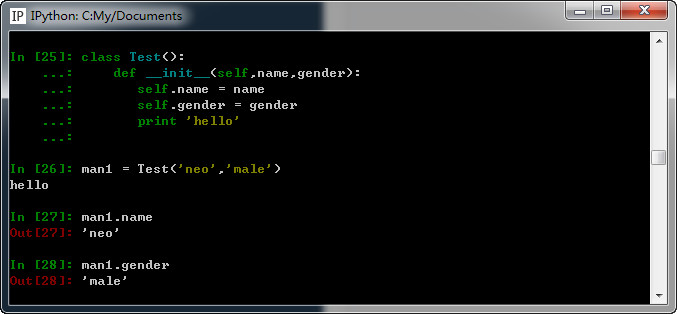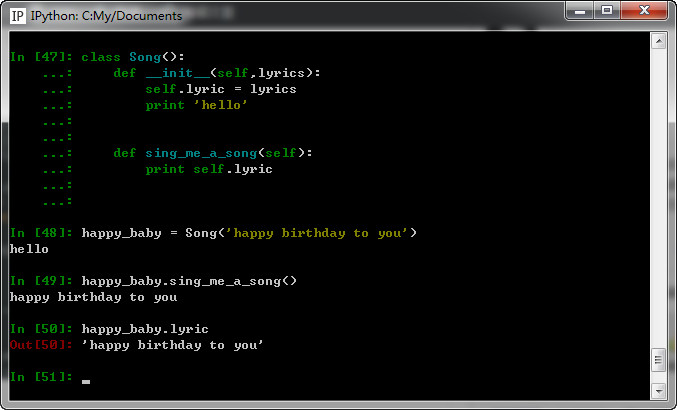Home >  > Python __init__ 用法

## Python __init__ 用法

0

```class Rectangle():
def getPeri(self,a,b):
return (a + b)*2
def getArea(self,a,b):
return a*b

rect = Rectangle()
print(rect.getPeri(3,4))
print(rect.getArea(3,4))
print(rect.__dict__)
```

```14
12
{}
```

```class Rectangle():
def __init__(self,a,b):
self.a = a
self.b = b
def getPeri(self):
return (self.a + self.b)*2
def getArea(self):
return self.a * self.b

rect = Rectangle(3,4)
print(rect.getPeri())
print(rect.getArea())
print(rect.__dict__)
```

```14
12
{'a': 3, 'b': 4}
```

__init__ 的意思是：initialize，初始化，因此可以理解实例化出某个类的对象时调用的初始化方法。

1、它位于类内部，可以让你在里面先做一些初始化工作，例如定义一些变量供后面的其它方法使用。

```class Song():
def __init__(self,lyrics):
self.lyric = lyrics

def sing_me_a_song(self):
for line in self.lyric:
print line

happy_baby = Song(['happy birthday to you',
'I do not want to get sued',
'So i will stop right there'])

bulls_on_parade = Song(['They rally around the family',
'with pockets full of shells'])

happy_baby.sing_me_a_song()